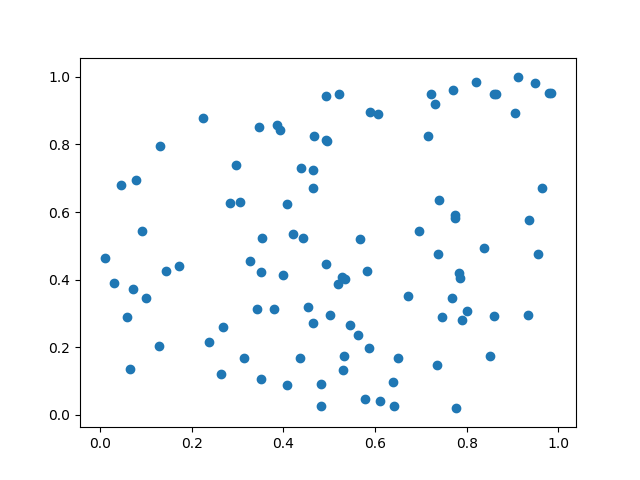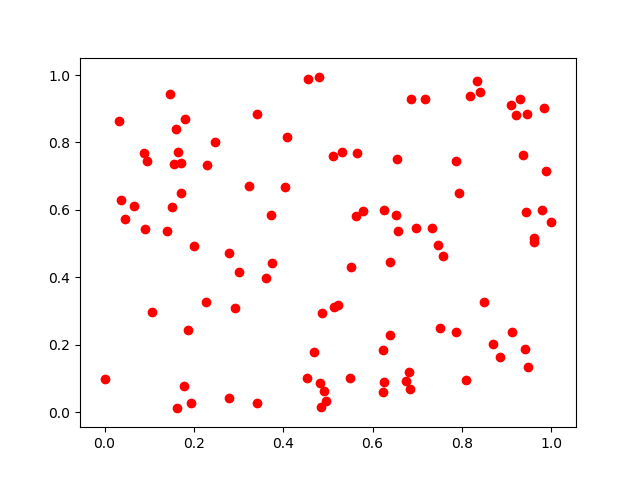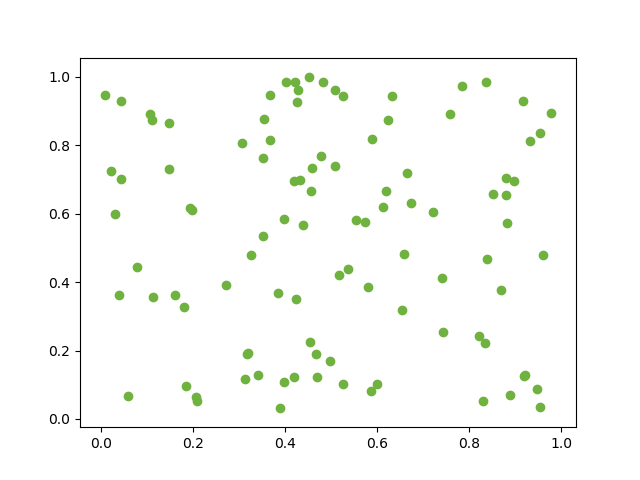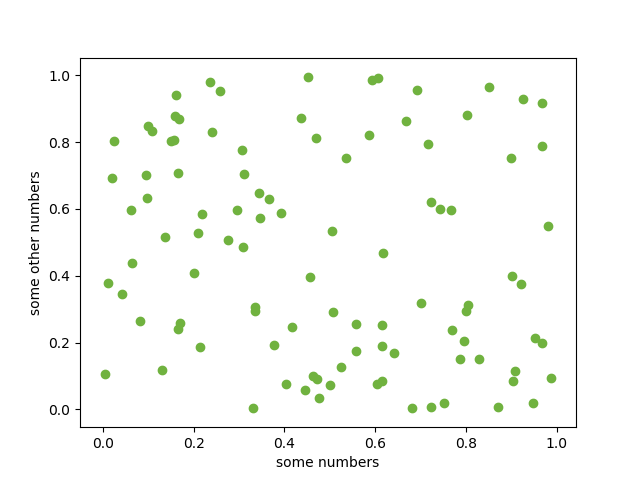When you area already familiar with the basic plot from the introduction to matplotlib here is another type of plot used in data science.

A very basic visualization is the scatter plot:

```import numpy as np
import matplotlib.pyplot as plt

N = 100
x = np.random.rand(N)
y = np.random.rand(N)

plt.scatter(x, y)
plt.show()```## Color of the dots

can be changed with the color parameter. The default is ‘b’ for blue. I f you want e.g. red the color just use ‘r’.

`plt.scatter(x, y, color="r")`The following table shows the basic colors

Color shortcut
blue b
green g
red r
cyan c
magenta m
yellow y
black k (not so obvious :-))
white w

In /matplotlib/_color_data.py you find additional colors even colors from XKCD color survey

`plt.scatter(x, y, color="xkcd:nasty green")`## Axis Labels```plt.ylabel('some other numbers')
plt.xlabel('some numbers')```